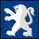94501 visualizzazioni
VFI,introduced by Markos Katsanos, is based on the popular On Balance Volume (OBV) but with three very important modifications:

* Unlike the OBV, indicator values are no longer meaningless. Positive readings are bullish and negative bearish .
* The calculation is based on the day's median (typical price) instead of the closing price.
* A volatility threshold takes into account minimal price changes and another threshold eliminates excessive volume .

A simplified interpretation of the VFI is:
* Values above zero indicate a bullish state and the crossing of the zero line is the trigger or buy signal.
* The strongest signal with all money flow indicators is of course divergence.

I have exposed options to plot a signal EMA . All parameters are configurable.

Markos suggests using 0.2 coeff for day trading and 0.1 for intra-day.

```//
// @author LazyBear
//
// If you use this code in its original/modified form, do drop me a note.
//

study(title = "Volume Flow Indicator [LazyBear]", shorttitle="VFI_LB")

length = input(130, title="VFI length")
coef = input(0.2)
vcoef = input(2.5, title="Max. vol. cutoff")
signalLength=input(5)
smoothVFI=input(false, type=bool)

ma(x,y) => smoothVFI ? sma(x,y) : x

typical=hlc3
inter = log( typical ) - log( typical )
vinter = stdev(inter, 30 )
cutoff = coef * vinter * close
vave = sma( volume, length )
vmax = vave * vcoef
vc = iff(volume < vmax, volume, vmax) //min( volume, vmax )
mf = typical - typical
vcp = iff( mf > cutoff, vc, iff ( mf < -cutoff, -vc, 0 ) )

vfi = ma(sum( vcp , length )/vave, 3)
vfima=ema( vfi, signalLength )
d=vfi-vfima

plot(0, color=gray, style=3)
showHisto=input(false, type=bool)
plot(showHisto ? d : na, style=histogram, color=gray, linewidth=3, transp=50)
plot( vfima , title="EMA of vfi", color=orange)
plot( vfi, title="vfi", color=green,linewidth=2)
```
List of my free indicators: http://bit.ly/1LQaPK8
List of my indicators at Appstore: http://blog.tradingview.com/?p=970

## CommentiSo far, just from a glance, the Accumulation Swing Index Oscillator seems to be the best from the bunch:
Rispondimika2k1
It has to be, since ASIOsc is my own concoction ;) j/k.

Thanks for the info, BTW.
Rispondi